Latest Banking jobs   »

# Reasoning Ability Quiz For IBPS RRB PO Clerk Mains 2022- 31st August

Directions (1-4): In every question two rows are given and to find out the resultant of a particular row you need to follow the given steps:

Step I: If an odd number is followed by an even number, then the resultant will be the multiplication of two numbers.
Step II: If an even number is followed by an odd number, then the resultant will be the absolute difference of the two numbers.
Step III: If an odd number is followed by an odd number (but not prime numbers), then the resultant will sum of the square of tens digit of both the numbers (in a number 75-5 is the unit digit and 7 is the tens digit)
Step IV: If an even number is followed by an even number, then the resultant will be the cube of the unit digit of the bigger number of them (in a number 75-5 is the unit digit and 7 is the tens digit).
Step V: If an odd number is followed by a prime number except 2, then the resultant will be the number formed by those two numbers i.e., 1st number is written besides the second number.
For e.g., if 1st number is 3 and the 2nd number is 5. So, the resultant will be 35.

Q1. What will be the sum of the resultant of both the rows?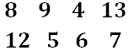(a) 44
(b) 52
(c) 92
(d) 08
(e) None of these

Q2. What is the resultant of row 2, if A is the resultant of Row 1?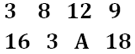(a) 288
(b) 128
(c) 271
(d) 216
(e) None of these

Q3. What will be the sum of the unit digits of the resultants of both the rows?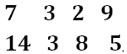(a) 13
(b) 10
(c) 15
(d) 18
(e) None of these

Q4. What is the resultant if we multiply 2 in resultant of the row given below?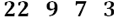(a) 2122
(b) 122
(c) 1886
(d) 2458
(e) None of these

Directions (5-7): In every question two rows are given and to find out the resultant of a particular row you need to follow the following steps:

Step I: If even number is followed by the number divisible by 3 then the resultant will be the addition of 4 with the difference of both the numbers.
Step II: If even number is followed by the number not divisible by 3 then the resultant will be smallest number among the two numbers.
Step III: If odd number is followed by a prime number, then the resultant will be the addition of both the numbers.
Step IV: If prime number is followed by any number (but not prime), then the resultant will be the difference of greater number with 5.
Step V: If odd number is followed by any number (both are not prime numbers), then the resultant will be the difference of both the numbers.

Q5. What is the difference of resultant of both the rows?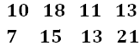(a) 9
(b) 10
(c) 11
(d) 2
(e) None of these

Q6. What is the resultant of first row if X is the resultant of second row?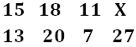(a) 5
(b) 9
(c) 12
(d) 15
(e) None of these

Q7. What is the sum of resultant of both the rows?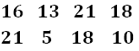(a) 13
(b) 14
(c) 15
(d) 16
(e) None of these

Directions (8-11): There are two rows given and to find out the resultant of a particular row, we need to follow the particular steps:

Step I: If an even number is followed by an odd number, then resultant will be the sum of both the numbers.
Step II: If an odd number is followed by an even number, then the resultant will be the difference of both the numbers.
Step III: If an odd number is followed by a square number (but not even number), then the resultant will be the multiplication of both the numbers.
Step IV: If an even number is followed by another even number which is greater than 10, then the resultant will be the difference of both the numbers.

Q8. What is the difference of resultant of both the rows?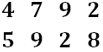(a) 56
(b) 60
(c) 64
(d) 62
(e) None of these

Q9. What will be the multiplication of resultant of both the rows?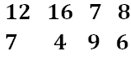(a) 26
(b) 48
(c) 63
(d) 76
(e) None of these

Q10. What is the sum of resultant of the both the rows?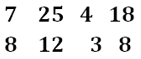(a) 156
(b) 153
(c) 158
(d) 154
(e) None of these

Q11. What will be the resultant of row I, if B is the resultant of row II?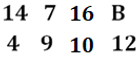(a) 87
(b) 45
(c) 59
(d) 95
(e) 42

Directions (12-15): In every question two rows are given and to find out the resultant of a particular row you need to follow the given steps:

Step I: If an odd number is followed by an even number, then the resultant will be the sum of both numbers.
Step II: If an even number is followed by an odd number, then the resultant will be the absolute difference of the both numbers.
Step III: If an odd number is followed by odd number, then the resultant will sum of the squares of both numbers.
Step IV: If an even number is followed by an even number, then the resultant will be the square of the tens digit of the smaller number of them (in a number 34-4 is the unit digit and 3 is the tens digit).

Q12. What is the sum of resultant of the both the rows?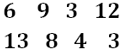(a) 635
(b) 636
(c) 645
(d) 656
(e) None of these

Q13. What is the half of the difference of resultant of both the rows?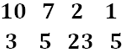(a) 65
(b) 63
(c) 60
(d) 50
(e) None of these

Q14. If we multiply the resultant of row 1 by 2 then, what is the difference of resultant of both the rows?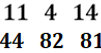(a) 9
(b) 7
(c) 10
(d) 12
(e) None of these

Q15. What will be the multiplication of resultant of both the rows?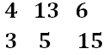(a) 288
(b) 298
(c) 169
(d) 285
(e) None of these

Solutions

S1. Ans. (a)
Sol. In row 1, according to the given step, resultant of row 1 is 9.
In row 2, according to the given step, resultant of row 2 is 35.
Hence, required sum = 9 + 35 = 44.

S2. Ans. (d)
Sol. In row 1, according to the given step, resultant of row 1 is 55. So, A =55
In row 2, according to the given step, resultant of row 2 is 216.

S3. Ans. (b)
Sol. In row 1, according to the given step, resultant of row 1 is 137.
In row 2, according to the given step, resultant of row 2 is 83.
Hence, required sum of the unit digits = 7 + 3 = 10.

S4. Ans. (e)
Sol. According to the given step, resultant of row is 1373.
So, after multiplying the resultant by 2 we get 2746.

S5. Ans. (a)
Sol. In row 1, according to the given step, resultant of row 1 is 24.
In row 2, according to the given step, resultant of row 2 is 15.
Hence, required difference = 24 – 15 = 9.

S6. Ans. (b)
Sol. In row 2, according to the given step, resultant of row 2 is 9. So, X = 9
In row 1, according to the given step, resultant of row 1 is 9.

S7. Ans. (d)
Sol. In row 1, according to the given step, resultant of row 1 is 6.
In row 2, according to the given step, resultant of row 2 is 10.
Hence, required sum = 6 + 10 = 16.

S8. Ans. (d)
Sol. In row 1, according to the given step, resultant of row 1 is 97.
In row 2, according to the given step, resultant of row 2 is 35.
Hence, required difference = 97 – 35 = 62.

S9. Ans. (c)
Sol. In row 1, according to the given step, resultant of row 1 is 3.
In row 2, according to the given step, resultant of row 2 is 21.
Hence, required multiplication = 3 x 21 = 63.

S10. Ans. (d)
Sol. In row 1, according to the given step, resultant of row 1 is 153.
In row 2, according to the given step, resultant of row 2 is 1.
Hence, required sum = 153 + 1 = 154.

S11. Ans. (b)
Sol. In row 2, according to the given step, resultant of row 2 is 9. So, B = 9.
So, according to the given step, resultant of row 1 is 45.

S12. Ans. (a)
Sol. In row 1, according to the given step, resultant of row 1 is 1.
In row 2, according to the given step, resultant of row 2 is 634.
Hence, required sum = 1 + 634 = 635.

S13. Ans. (c)
Sol. In row 1, according to the given step, resultant of row 1 is 26.
In row 2, according to the given step, resultant of row 2 is 146.
So, difference = 146 – 26 = 120. Hence, half of the 120 is 60.

S14. Ans. (b)
Sol. In row 1, according to the given step, resultant of row 1 is 29.
After multiplication it is 58.
In row 2, according to the given step, resultant of row 2 is 65.
So, required difference = 65 – 58 = 7.

S15. Ans. (d)
Sol. In row 1, according to the given step, resultant of row 1 is 15.
In row 2, according to the given step, resultant of row 2 is 19.
So, required multiplication = 15 x 19 = 285.#### Congratulations!Download Hindu Review of October 2021: Free PDF